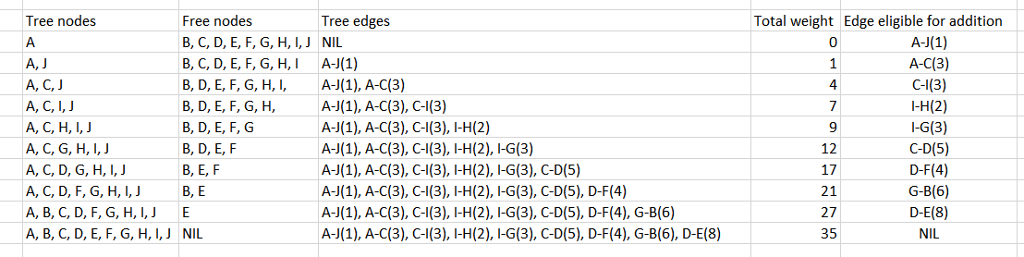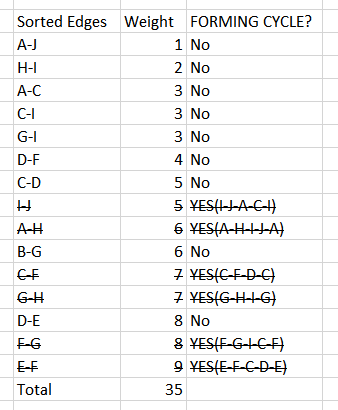# Solved: Find the MST using a) Kruskal’s algorithm and b) Prim’s algorithm

Find the MST using a) Kruskal’s algorithm and b) Prim’s algorithm

(10 points each). Show each step of the procedure and what’s the minimum cost?

Don't use plagiarized sources. Get Your Custom Essay on
Solved: Find the MST using a) Kruskal’s algorithm and b) Prim’s algorithm
GET AN ESSAY WRITTEN FOR YOU FROM AS LOW AS \$13/PAGE

B.     Show the order in which vertices are visited using a) DFS and

b) BFS (10 points each). Edges that are given are undirected.

C) Give a topological sort (10 points).

Assume     ‘<‘ = ‘->’.

D.     Summary report on 2 of the 3 algorithms (Dijkstra’s, Bellman-Ford’s, Floyd-Warshall’s)

User the table below and assume the graph is undirected, please draw the graph and show each step

 A B C D E F G H I J A 0 0 3 0 0 0 0 6 0 1 B 0 0 0 0 0 6 0 0 0 C 0 5 0 7 0 0 3 0 D 0 8 4 0 0 0 0 E 0 9 0 0 0 0 F 0 8 0 0 0 G 0 7 3 0 H 0 2 0 I 0 5 J 0

Based on the guideline, I can only solve a and b subpart of 1st question. I can at most upload the DFS and BFS for you upon request

PRIMS:

Let us start from A… So we add A to the empty tree
Step 1: We add the shortest route from A to X such that X is not in the tree

Step 2: Edge A-J is added and J is included in the Tree since J has the minimum weight

Step 3: We add the shortest route from A or J to X such that X is not in the tree
Step 4: We add A-C with weight 3 and C is added in the tree

Step 5: We add the shortest route from A or C or J to X such that X is not in the tree
Step 6: We add edge C-I with weight 3 and I is added in the tree

Step 7: We add the shortest route from A or C or I or J to X such that X is not in the tree
Step 8: We add edge I-H with weight 2 and H is added in the tree

Step 9: We add the shortest route from A or C or H or I or J to X such that X is not in the tree
Step 10: We add edge I-G with weight 3 and G is added in the tree

Step 11: We add the shortest route A,C,G,H,I,J to X such that X is not in the tree
Step 12: We add edge C-D with weight 5 and D is added in the tree

Step 11: We add the shortest route A,C,D,G,H,I,J to X such that X is not in the tree
Step 12: We add edge D-F with weight 4 and F is added in the tree

Step13: We add the shortest route A,C,D,G,H,I,J to X such that X is not in the tree
Step 14: We add edge G-B with weight 6 and B is added in the tree

Step 15: We add the shortest route A,BC,D,G,H,I,J to X such that X is not in the tree
Step 16: We add edge D-E with weight 8 and D is added in the treeKruskal:

Step1: arrange edges in accending order of their weights
Step2: Add edges starting from smallest weight to largest weight unless they form cyclel ike the example: